Classically the bent beam equation has mostly assumed small first derivatives of vertical displacement with respect to the distance along the beam. With that assumption the differential equations are linear. We solve and plot some cases numerically here without that assumption.

Consider a thin beam bent in 2 dimensions so that the torque at a point is proportional to its curvature, which is the commonest assumption. We assume that the beam is at rest and in equilibrium, perhaps unstable. In a plane, the torque is a linear function of the position and we assume here that the curvature at point <x, y> is Ty where T is an elastic parameter that is constant along the beam in these calculations. We normalize by assuming that the curve passes thru <0, 1> parallel to the X axis there. What is the shape of this curve?

It is easy to compute the curve if we take its arc length, τ, as the independent variable and consider x, y and α as dependent variables. α is the angle, measured counter-clockwise from the positive X direction, in radians, of the tangent to the curve. The initial conditions are

• τ = 0
• α = 0
• x = 0
• y = 1
The differential equations are
• dα/dτ = −Ty
• dx/dτ = cos α
• dy/dτ = sin α
We describe a one parameter family of curves—T is the parameter.
This too simple Java code, controlled by this makes the following pictures:

java Main 1 0: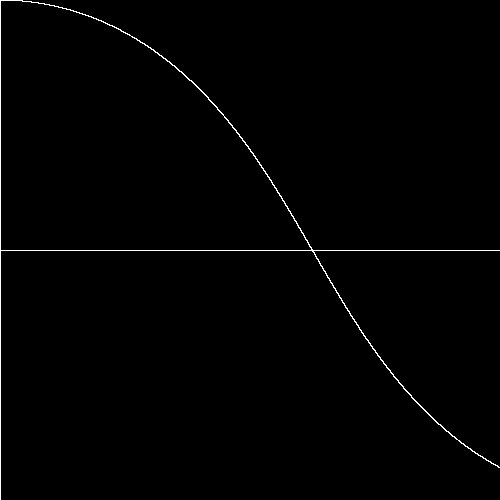java Main 2 0: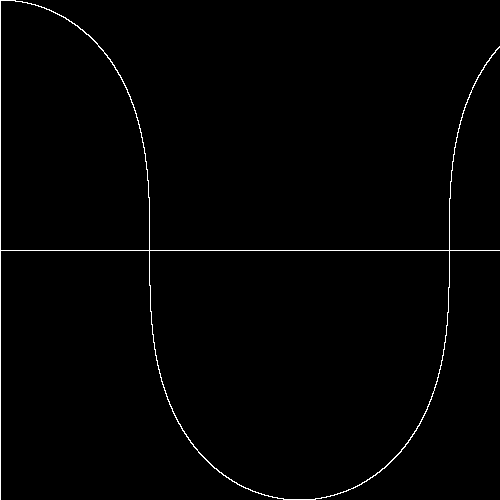java Main 2.5 0: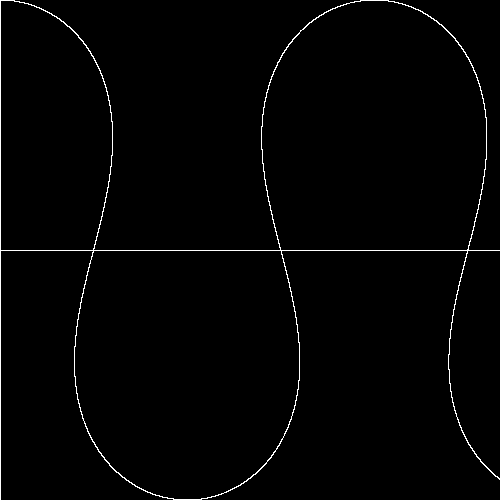java Main 3 0: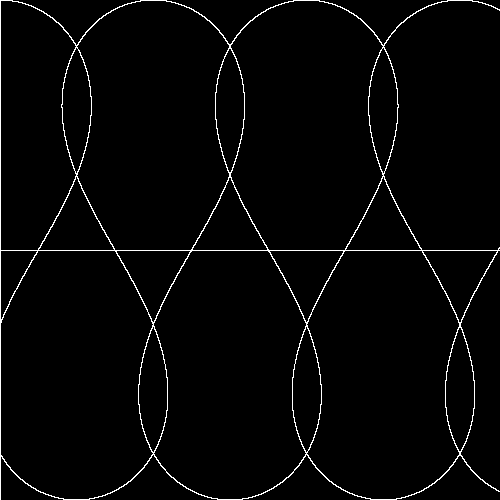java Main 3.30373 1.0: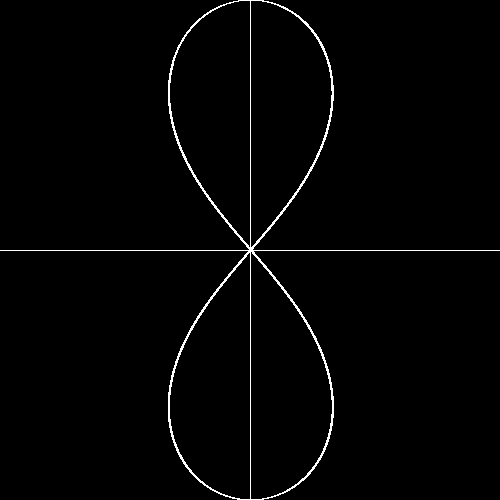Alas not a lemniscate!!
java Main 3.5 1.7: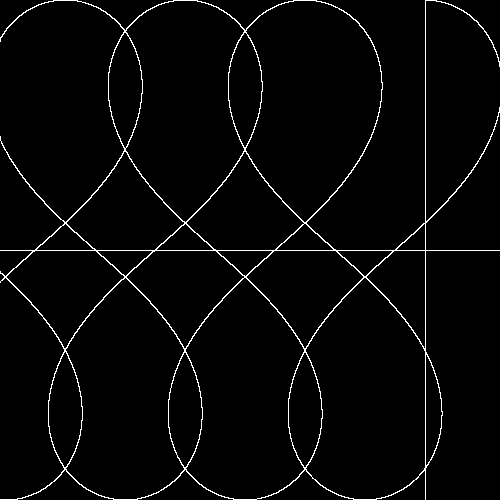java Main 3.9 1.7: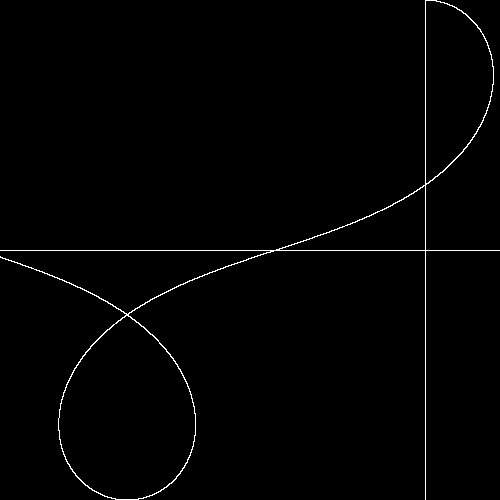java Main 4.0 1.7: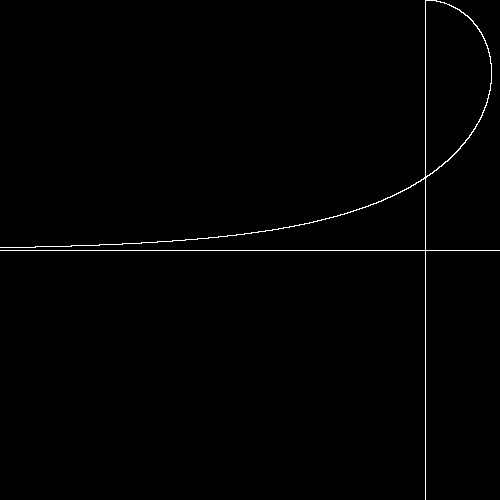Alas not a Folium
java Main 4.2 1.7: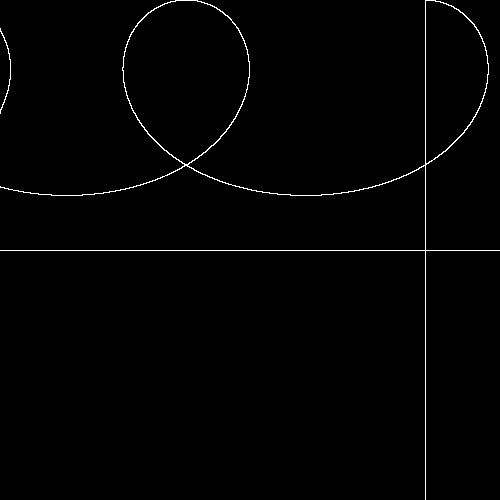java Main 4.4 1.7: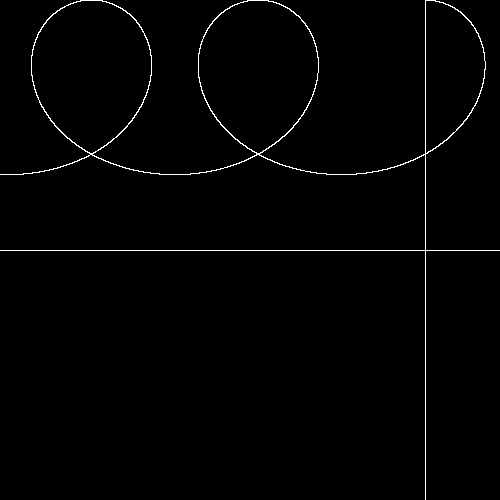java Main 5 1.7: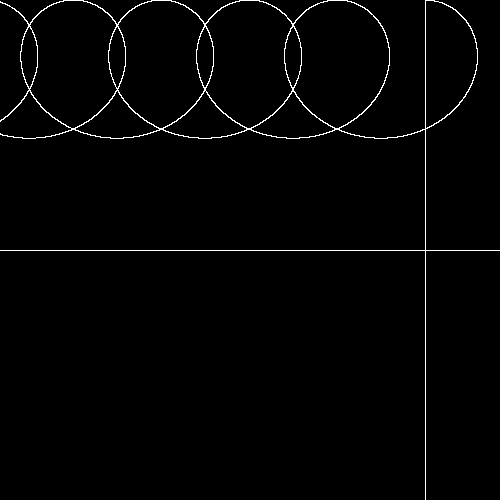In this family of curves there is a close mimic of the Folium of Descartes rotated by π/4 when T = 4, but it is not the same. When T is about 3.30373 it is nearly, but not quite a lemniscate.

For negative values of T we get a rescaled reprise of the curves from T > 4. These curves bounded away from the X axis—indeed y ≥ 1.